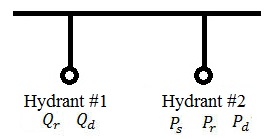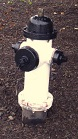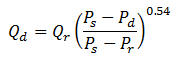Fire Hydrant Flow Test Analysis

Compute hydrant residual flow, pressures

Register to enable "Calculate" button in fire hydrant flow test calculator.

 Compute Ps Compute Pr Compute Pd Compute Qr Compute Qd Static Pressure, Ps : psi N/m2 mbar bar Residual Pressure, Pr : psi Desired Pressure, Pd : psi LMNO Engineering www.LMNOeng.com Flow Qr at Pr : cfs gpm liter/s liter/min m3/s Flow Qd at Pd : gpm

Plan View of Portion of Pipe System Containing Fire Hydrants and Photo of a Hydrant in Athens, OhioFire Hydrant Flow Test Analysis Equation (AWWA, 2006, p. 52)Fire Hydrant Flow and Pressure Tests
Fire hydrants are tested to determine flow and pressure. Referring to the figure, prior to flowing hydrant #1, pressure is measured at hydrant #2. This is the static pressure Ps. Then hydrant #1 is opened so that water discharges from it. When the flow rate out of hydrant #1 is relatively steady, the pressure at hydrant #2 is measured. This is called the residual pressure Pr. At nearly the same time, the flow rate out of hydrant #1 is recorded. This is the flow rate Qr at residual pressure Pr. After making the three measurements Ps, Pr, and Qr, the test is over and the fire hydrants are slowly closed. It is best if Ps-Pr is at least 10 psi (0.69 bar) (AWWA, 2006, p. 45). Further details of how to conduct a fire hydrant test can be found in the American Water Works Association publications listed below.

After conducting the fire hydrant test, the calculator above can be used to determine the flow rate Qd at the desired pressure Pd. The calculator uses the equation shown above. The desired pressure Pd is typically 20 psi. For instance, if the static pressure Ps was measured as 68 psi, the residual pressure Pr was measured as 43 psi, and the flow Qr at the residual pressure was measured as 1710 gpm, then the flow Qd at the desired pressure of 20 psi is 2432 gpm using the calculator. The flow rate Qd of 2432 gpm is higher than the measured value Qr of 1710 gpm. If a fire hose with a pump is connected to hydrant #1 and the pump turned on drawing water out of the hydrant, the effect of drawing water out of the hydrant will cause the pressure at hydrant #2 to drop. If the pressure in hydrant #2 drops to Pd of 20 psi, then the expected flow rate out of hydrant #1 is Qd of 2432 gpm.

The computation of the desired flow Qd is based on the equation above which is the most commonly used equation for fire hydrant flow test analysis. The equation has a coefficient of 0.54 which is based on the Hazen-Williams equation (see Hazen-Williams Equation or Hazen-Williams Design Calculator). The Hazen-Williams equation is widely used to analyze municipal water flow in pressurized pipes. For modeling pressurized pipe flow, the Hazen-Williams equation includes pipe diameter, pipe length, and a pipe roughness coefficient. None of those parameters are in the fire hydrant test equation. Since the fire hydrant equation does not include those parameters, the results of a particular hydrant test cannot be expected to necessarily predict test results of other hydrants. Also, since other factors impact performance of the pipe system, tests at other times or other days will likely yield different measured values for Ps, Pr, and Qr. Homes, businesses, industries, and other water users have different water demands on the piping system from one day to another and from time to time within a day. Also, a water system may get expanded, pipes may get cleaned, or pipes may get calcified.

In addition to computing the flow rate Qr at desired pressure Pr, you can solve for any of the five parameters by selection from the drop-down menu in the calculator.

Units in fire hydrant flow test analysis calculation
cfs=cubic foot per second, gpm=US gallon per minute, m=meter, mbar=millibar, N/m2=Newton per square meter (Pascal), psi=pound per square inch, s=second

Error Messages given by fire hydrant flow and pressure test calculator
Input checks:
"Invalid input": Most likely a non-numeric character was entered such as a letter or a space.
"Need Ps > 0", "Need Pr > 0", "Need Pd > 0", "Need Qr > 0", "Need Qd > 0", "Need Ps > Pr".
"Cannot enter Qd = Qr" if solving for Ps.
"Cannot enter Pd = Pr" if solving for Ps.
Run-time errors:
"Ps will be ≤ 0", "Ps will be ≤ Pr", "Ps will be ≤ Pd", "Pr will be ≤ 0", "Pd will be ≤ 0".

References
American Water Works Association [AWWA]. (2005). Computer modeling of water distribution systems. Manual of Water Supply Practices. M32. 2nd edition.

American Water Works Association [AWWA]. (2006). Installation, field testing, and maintenance of fire hydrants. Manual of Water Supply Practices. M17. 4th edition.

© 2018 LMNO Engineering, Research, and Software, Ltd.  All rights reserved.

Please contact us for consulting or other questions.

LMNO Engineering, Research, and Software, Ltd.
7860 Angel Ridge Rd.   Athens, Ohio  45701  USA   Phone: (740) 592-1890
LMNO@LMNOeng.com    https://www.LMNOeng.com

To:
LMNO Engineering home page (all calculations)

Related calculations:

Unit Conversions

Register